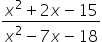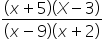# CAT 2021 Slot 1

## CAT 2021 Exam Question Papers Slot 1 | CAT Quants​

### CAT Quantitative Aptitude | CAT 2021 Question Papers​

CAT Question Papers: Solving CAT Question Papers from prior years will give CAT 2022 applicants invaluable insight into the examination format, question pattern, and level of difficulty. The CAT 2021 exams were held in three slots.

It is important for CAT preparation to examine old CAT Exam, Question Paper. In order to overcome the difficulties, they encounter when preparing for the CAT, aspirants must use a variety of strategies.
• By solving CAT Exam previous year question papers, aspirants learn about their own strengths and weaknesses. This allows them to plan their preparation accordingly.
• The secret to passing any exam is practice. Hence, by studying CAT exam papers over the last 5 to 10 years, you can attempt 500+ questions of all types and levels of difficulty.
• They can identify important topics by asking questions that have been asked repeatedly over time.
• CAT aspirants can benefit from numerous study materials and engaging video lessons available at Pathfinder For Me, including Free Download CAT Exam E-Books. Aspirants for the CAT test will get closer to passing the CAT 2022 exam with the help of these CAT question papers with solution PDF.

The best resource for CAT preparation is CAT question papers. Solving previous year’s CAT question papers helps candidates understand the CAT Exam Pattern, types of questions, question weightage, important topics, etc.

All CAT 2021 Quantitative aptitude questions have detailed answer keys and solutions provided by our experts. Watch the videos below to learn the fastest ways to answer the questions:

3).

f(x) =is negative if and only if

1. -5 < x < -2 or 3 < x < 9

2. -2 < x < 3 or x > 9

3. x < -5 or 3 < x < 9

4. x < -5 or -2 < x < 3

### Explanation

By taking factors of numerator and denominator, the function can be written as

f(x) =The function will be negative if and only if one or three of the factors are negative.

When x < -5, all factors are negative. If -5 < x < - 2, only (x +5) is positive. If  3 < x < 9, only (x – 9) is negative.

Thus, f(x) =is negative if and only if  -5 < x < -2 or  3 < x < 9

Ans (1)